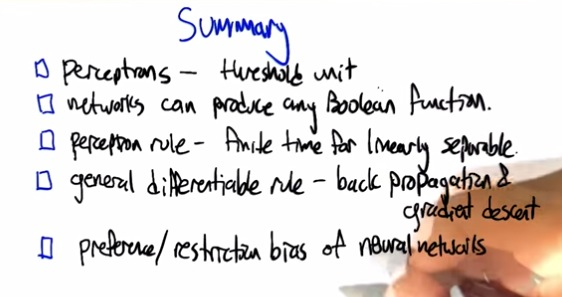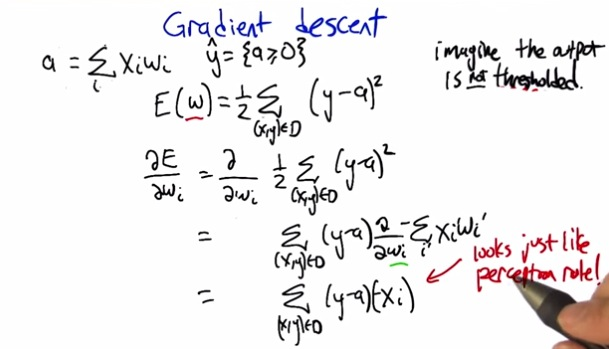• So the way gradient descent works is take a derrivative of the formula
• We take derrivative w.r.t weight parameters
• 1/2 is kept there, so when squared take derrivate, it will get nullified by 1/2
• then like derrivative, we kept the value, and the value derrivative.
• as activatios is just product of dataxweight, when we derrivative weight, weight will get nullified.
• So what's that left is the value, times data. Take into summation of each dataset(iterations)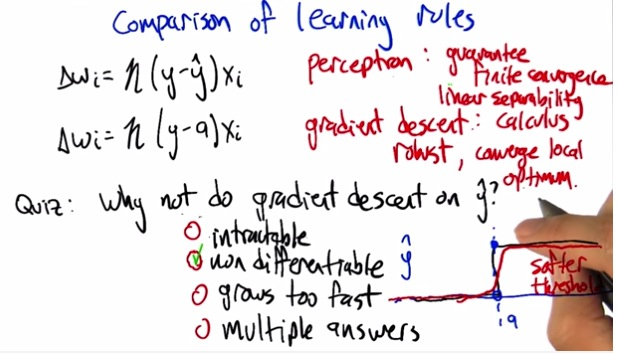• So perceptron always converge global minimum while gradient descent don't
• But gradient descent itself is not a threshold(non differentiable). We can't differentiate in gradient descent because it will have softer, curve. We can't differentiate using gradient descent if y using 1 or 0.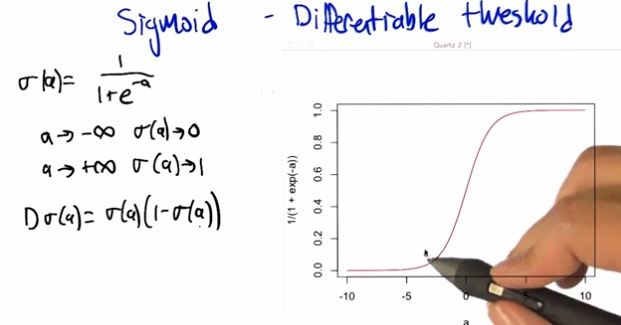• Here we have the sigmoid function to make activation to be differentiable
• What it's mean is that from the plot curve (s-ish/sigmoid) we know where activation can stand
• as a towards negative infinity sigmoid equals zero, On the contrary towards positive infinity will be equals to one.
• The final formula used to plot the curve so we know exactly where the differentiable value.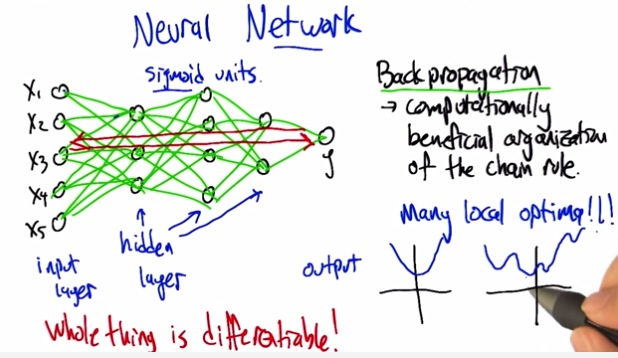• So this is the whole architecture of neural networks.
• There's unit, input layer, represent each of the attributes.
• Couple of hidden layer and output layer
• When we have gradient descent on one unit. It may converge to global minimum. But as we have many activation units performing gradient descent, we may end up at local optima
• The other advantage is the backpropagtion. Where one iterations, produce backpropagation from output, error as a weight of a next iterations. And then on and on until  final iterations.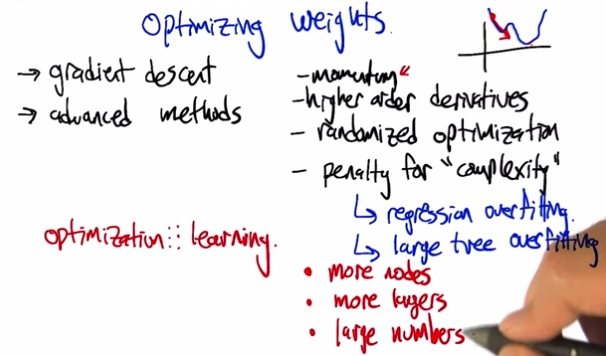• Now eventhough the gradient descent risk of local optima, there's also some optimization that we can use
• Momentum using physics-like gravity that eventhough we have local optima, it tries to bounce down to global minimum
• We can increase complexity, but beware also of the penalty.More nodes/layers/numbers also part of increasing complexity(variance)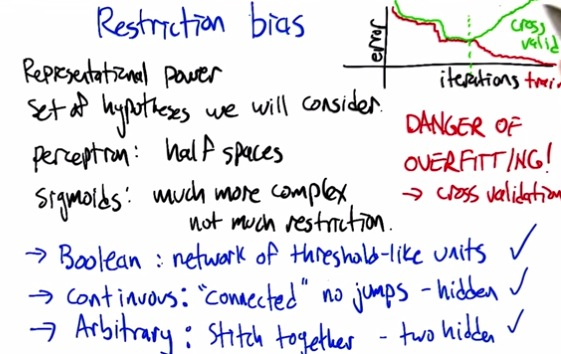• Here are the restriction bias for neural networks.
• We have boolean function, then use network of threshold
• If it continous, where's the case is no jumps, we can make one hidden units.
• But  if it's arbitrary, boolean/continuous, then we can combine together to make two hidden units.
• Careful as we increase hidden layer, we increase complexity and thus prone to overfitting.
• If we plot a curve, the error may go down as we train our model. But in cross-validation it may go down, then level up. From here we know as we take iterations of configuring our model, keep in mind of overfitting.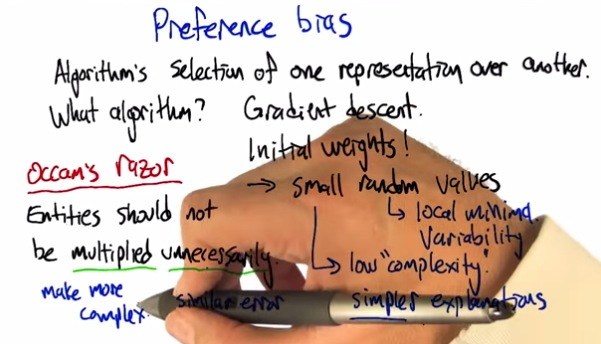• This is preferrence bias, where we decide what we prefer in algorithm to build our model.